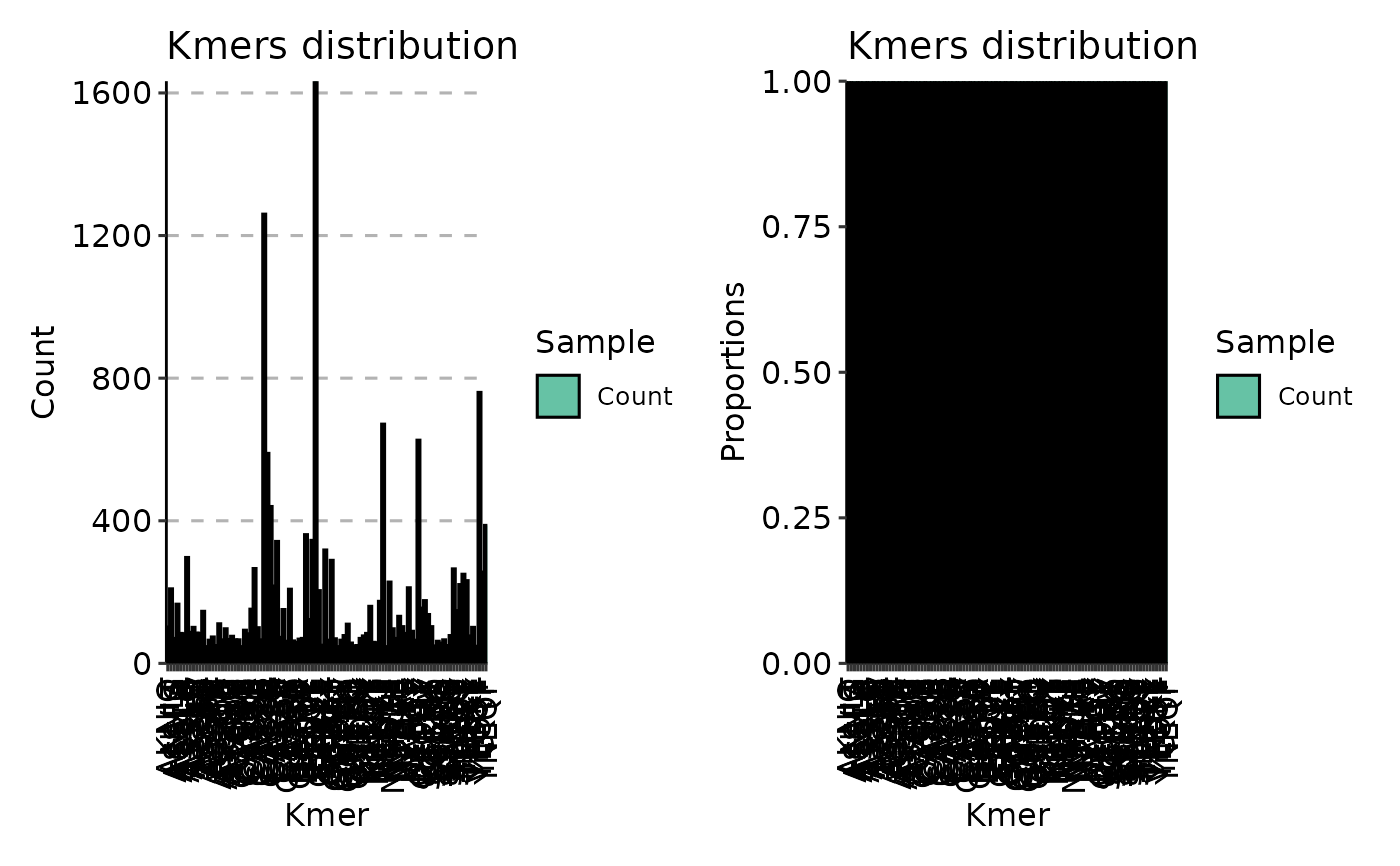Plot a distribution (bar plot) of the most frequent kmers in a data.

# S3 method for immunr_kmer_table
vis(
.data,
.position = c("stack", "dodge", "fill"),
.log = FALSE,
...
)

## Arguments

.data

Data frame with two columns "Kmers" and "Count" or a list with such data frames. See Examples.

Number of the most frequent kmers to choose for plotting from each data frame.

.position

Character vector of length 1. Position of bars for each kmers. Value for the ggplot2 argument position.

.log

Logical. If TRUE then plot log-scaled plots.

...

Not used here.

## Value

A ggplot2 object.

get.kmers

## Examples

# Load necessary data and package.
data(immdata)
# Get 5-mers.
imm.km <- getKmers(immdata\$data[], 5)
# Plots for kmer proportions in each data frame in immdata.
p1 <- vis(imm.km, .position = "stack")
p2 <- vis(imm.km, .position = "fill")
p1 + p2